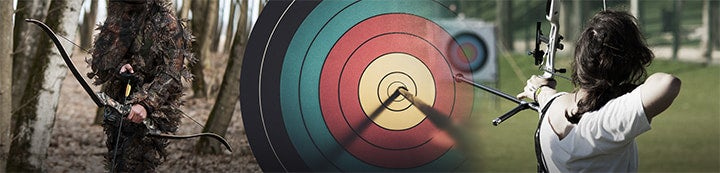1 - 8 of 8 Posts

#### Koala

·
##### Registered
Joined
·
42 Posts
Discussion Starter · ·
Anyone with information on how these two factors influence speed? How much speed do you gain by adding each pound of draw weight? How much speed do you gain as you lighten your arrows? Is there a consistent formula or do these factors change from bow setup to bow setup?

#### beezaur

·
##### Registered
Joined
·
2,425 Posts
The other factor is draw length.

You can invoke the work-energy principle (without even knowing what that means) to figure out how much energy is stored in the bow at full draw, then use an approximation of efficiency to estimate the speed.

(Oh Lord, here I go toward the math precipice again. . .)

PART 1: from scratch

To calculate how much energy is stored in your bow, you have to measure the draw force at regular intervals, say each inch. Then multiply the force times the incremental distance. For example, you might start off at 10# after the first inch. So you have 10 inch-pounds of energy stored in that first inch. Keep a running total until full draw - that is how many inch-pounds of energy the bow stores.

There are 12 inches in a foot, so divide by 12 to get the number of foot-pounds of stored energy.

Now assume you loose 60% of that energy to vibration and friction. So take 40% (a guess - each bow and draw weight will vary) of the bow's stored energy and assume the arrow comes off the rest with this much kinetic energy.

Remember the formula for energy (KE) given arrow weight in grains (w) and velocity in feet per second (v)? Just go backward:

KE = v^2 x w / 450,240

So. . .

v = sqrt( KE x 450,240 / w )

where v is the estimated velocity of the arrow, and 'sqrt()' is the square root.

PART 2: additional velocity per pound of draw

A very rough approximation of the extra energy stored over the entire length of the draw for each extra pound of draw weight is a half pound times the "power stroke." This will usually be around 20", so you can assume 10 inch-pounds of extra energy, or 10 / 12 = 0.833 ft-lbs per additional pound of draw weight with that particular case.

Anyway, just use 40% (or whatever) of the *extra* stored energy in the same equation. The velocity you get will be the extra velocity.

PART 3: the better way

Ask someone with a similar bow. These kinds of things can be very difficult to predict - too many unknowns!

Scott

PS: actually, it isn't that hopeless. If you know the efficiency, you have it beat.

#### NavyDMO

·
##### Registered
Joined
·
129 Posts
Riiiiiiiiight. What he said.

#### DeerForce1

·
##### Registered
Joined
·
212 Posts
Beezaur, can you spell that for us?I'm going to rad that a little closer in the morning when it's no longer the middle of the night.Koala, on average you will gain about 1 fps for each additional pound of draw weight & will gain 1 fps for every 5 grains of arrow weight you take off. These are averages for most bows, some may gain more some may not gain as much.............................................................DF1

#### Ian

·
##### X's R Us
Joined
·
3,458 Posts
About 2fps per lb of draw weight.

#### beezaur

·
##### Registered
Joined
·
2,425 Posts
Hehe, like I said, ask someone with a similar bowScott

#### Brown Hornet

·
##### Registered
Joined
·
34,167 Posts
I have found that it is usually about two feet for every pound of weight and every 5 grains you subtract from your arrow. But the easiest way to know is to shot through a cronograph.

#### Hollowpoint

·
##### Registered
Joined
·
5,455 Posts
My carbons weigh just a little over 100gr less than my aluminums and shoot 21fps faster.

1 - 8 of 8 Posts